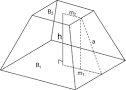## How to Calculate and Solve for the Height and Volume of a Square Pyramid Frustum | Nickzom CalculatorThe image above is a square pyramid frustum.

To compute the volume of a square pyramid frustum, three essential parameters are needed and this parameters are base side length (a), top side length (b) and height (h).

The formula for calculating the volume of a square pyramid frustum:

V = h(a² + ab + b²)3

Where;
V = Volume of a square pyramid frustum
a = Base side length of the square pyramid frustum
b = Top side length of the square pyramid frustum
h = Height of the square pyramid frustum

Let’s solve an example
Find the volume of a square pyramid frustum when the base side length is 10 cm with a top side length of 12 cm and a height of 20 cm.

This implies that;
a = Base side length of the square pyramid frustum = 10 cm
b = Top side length of the square pyramid frustum = 12 cm
h = Height of the square pyramid frustum = 20 cm

V = h(a² + ab + b²)3
V = 20(10² + (10 x 12) + 12²)3
V = 20(100 + (120) + 144)3
V = 20(364)3
V = 72803
V = 2426.6

Therefore, the volume of the square pyramid frustum is 2426.6 cm3.

Calculating the Height of a Square Pyramid Frustum when the Volume, Top Side Length and Base Side Length of the Square Pyramid Frustum is Given.

h = 3V / (a2 + ab + b2)

Where;
h = Height of the square pyramid frustum
V = Volume of the square pyramid frustum
a = Base side length of the square pyramid frustum
b = Top side length of the square pyramid frustum

Let’s solve an example;
Find the height of a square pyramid frustum when the volume is 32 cm3, base side length is 14 cm and top side length is 18 cm.

This implies that;
V = Volume of the square pyramid frustum = 32 cm3
a = Base side length of the square pyramid frustum = 14 cm
b = Top side length of the square pyramid frustum = 18 cm

h = 3V / (a2 + ab + b2)
h = 32 x 3 / (142 + (14 x 18) + 182)
h = 96 / (196 + 252 + 324)
h = 96 / 772
h = 0.124

Therefore, the height of the square pyramid frustum is 0.124 cm.